'); } })(); /*]]>*/'); } })(); /*]]>*/'); } else { document.write(''); } } })(); /*]]>*/'); document.write(''); document.write(''); if (/[?|&]ex-dp/.test(window.location.href) || document.cookie.indexOf('ex-dp=') !== -1) { bbcdotcom.utils.addHtmlTagClass('bbcdotcom-ex-dp'); } } })(); /*]]>*/'); } })( bbcdotcom.config.isOptimizelyEnabled() );

Five films that don't get school quite right - BBC Bitesize

# Five films that don't get school quite right

## Things are very different in British and American schools. But you don't need to have been educated Stateside to spot the inaccuracies that slip into so many Hollywood teen flicks, as well as British ones.

We can just about overlook the fact the kids are played by grown-ups, and that so many teachers are inspirational rule-breakers. Other blips though are harder to ignore.

Life at school does not revolve around cliques, sports, parties and the prom. Contrary to what movies suggest, kids spend more time at their desks than at their lockers. After all, they are there to pass exams. But how often do you see them doing homework? And does anyone in a coming of age movie have acne?

Here are five obvious slips.

## The Breakfast Club (1985)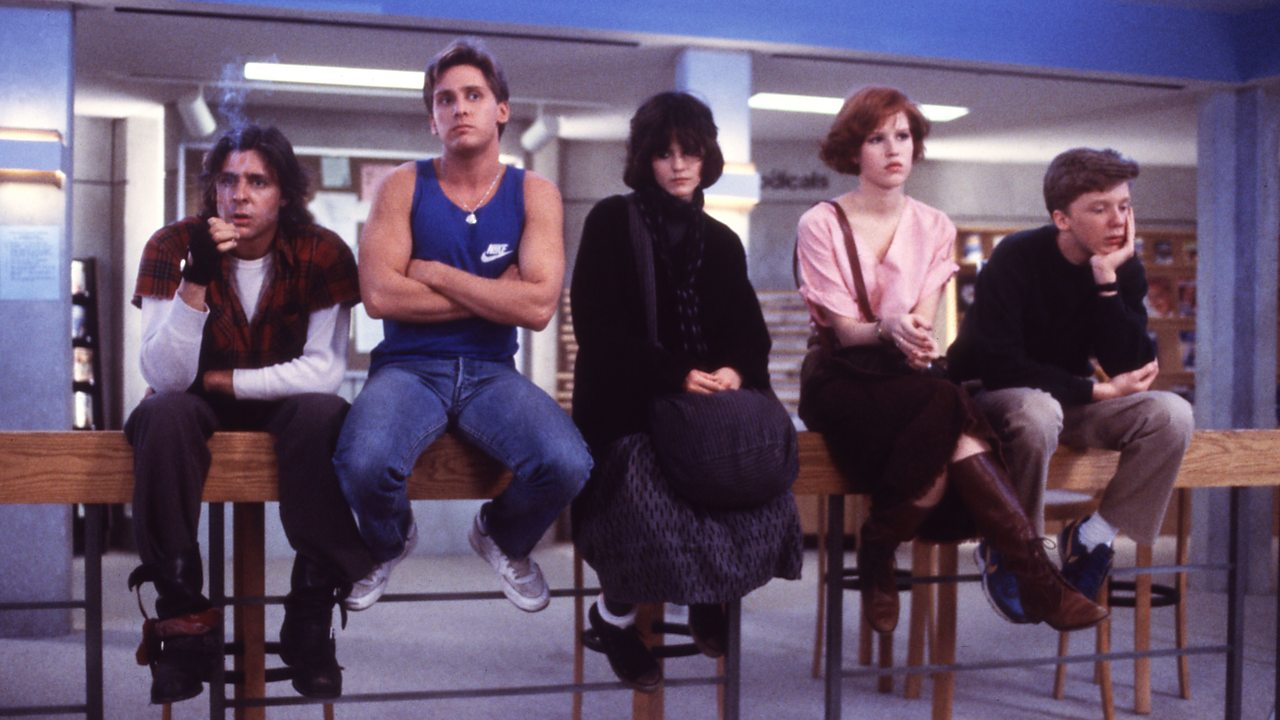"You see us as a brain, an athlete, a basket case, a princess and a criminal."

Anyone who has ever been in detention knows that a staff member is supposed to supervise the entire session. Yet, in John Hughes's magnum opus, assistant principal Richard Vernon (Paul Gleason), leaves the school library door open so he can keep an eye on the five students facing an all-day Saturday punishment from his office. Once Bender (Judd Nelson) sabotages the door, however, Vernon has no idea what the kids are doing. Of course, there wouldn't be a movie if he had simply done his job.

## Mean Girls (2004)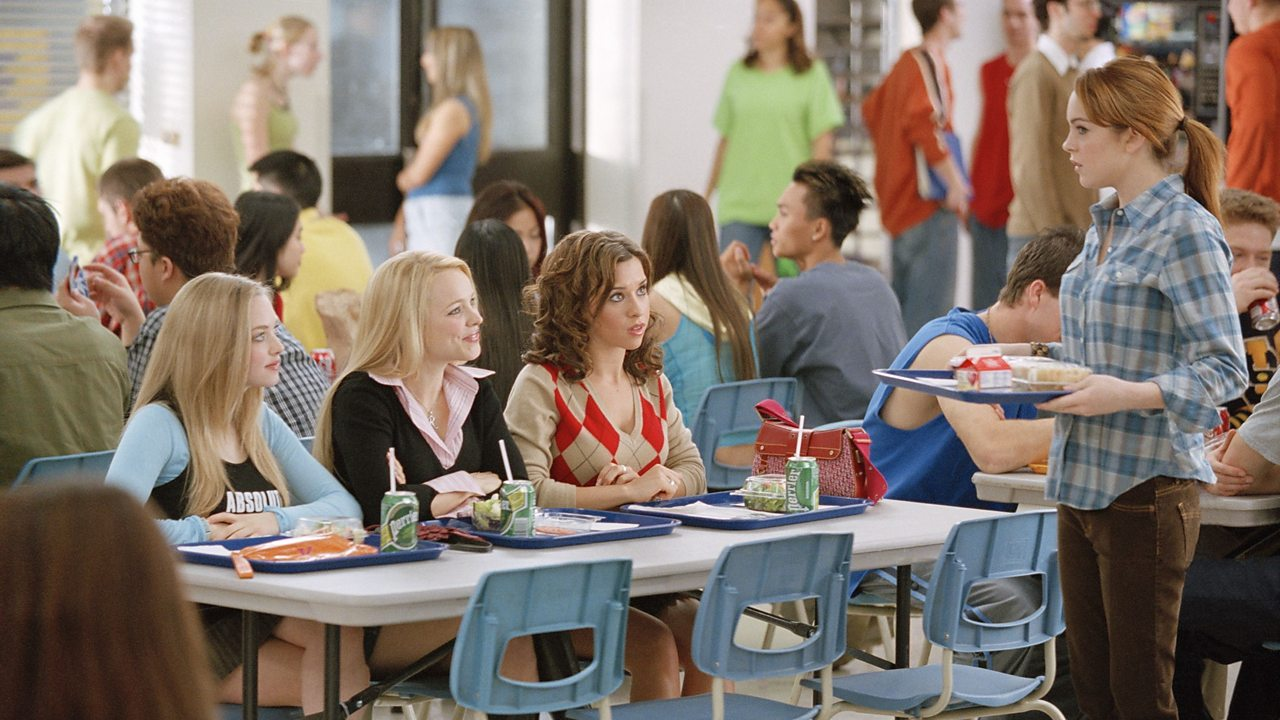"You can't sit with us!"

Friends usually try to sit together at lunchtime, but anyone who’s been to school knows it’s almost impossible to find a table. This makes the canteen map given to Cady Heron (Lindsay Lohan) in this Tina Fey-scripted rite of passage so unbelievable, as groups like the 'girls who eat their feelings' and the 'girls who don't eat anything' wouldn't get to sit at the same table each day in such a first-come, first-served environment. Besides, where do the non-clique kids sit?

## High School Musical (2006)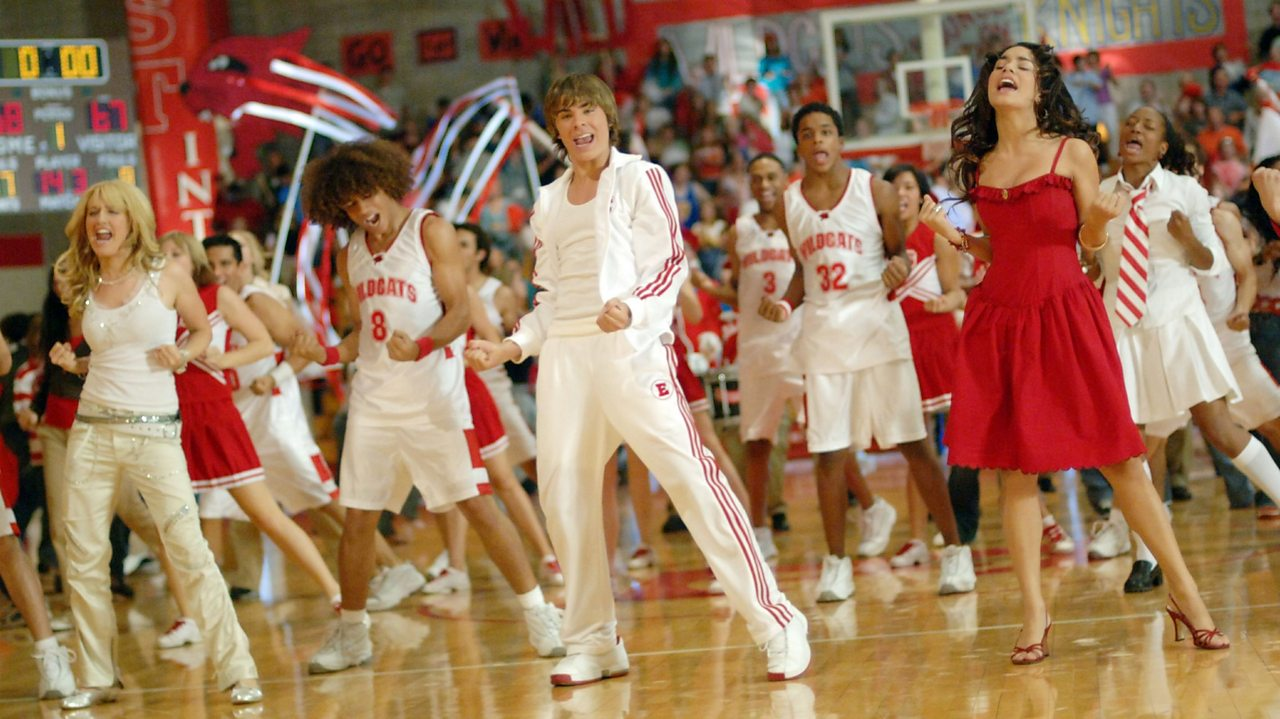"We're all in this together!"

Not many UK teenagers would spend their evenings and weekends supporting the school team. That's a job for proud parents. Sports are taken very seriously in US institutions, but the levels of school spirit are way off the chart during the East High Wildcats basketball game in Kenny Ortega's hit musical. It's one thing for the cheerleaders to know the words to the battle chant and all the dance moves, but everyone in the crowd is word and step perfect, too!

## The Inbetweeners Movie (2011)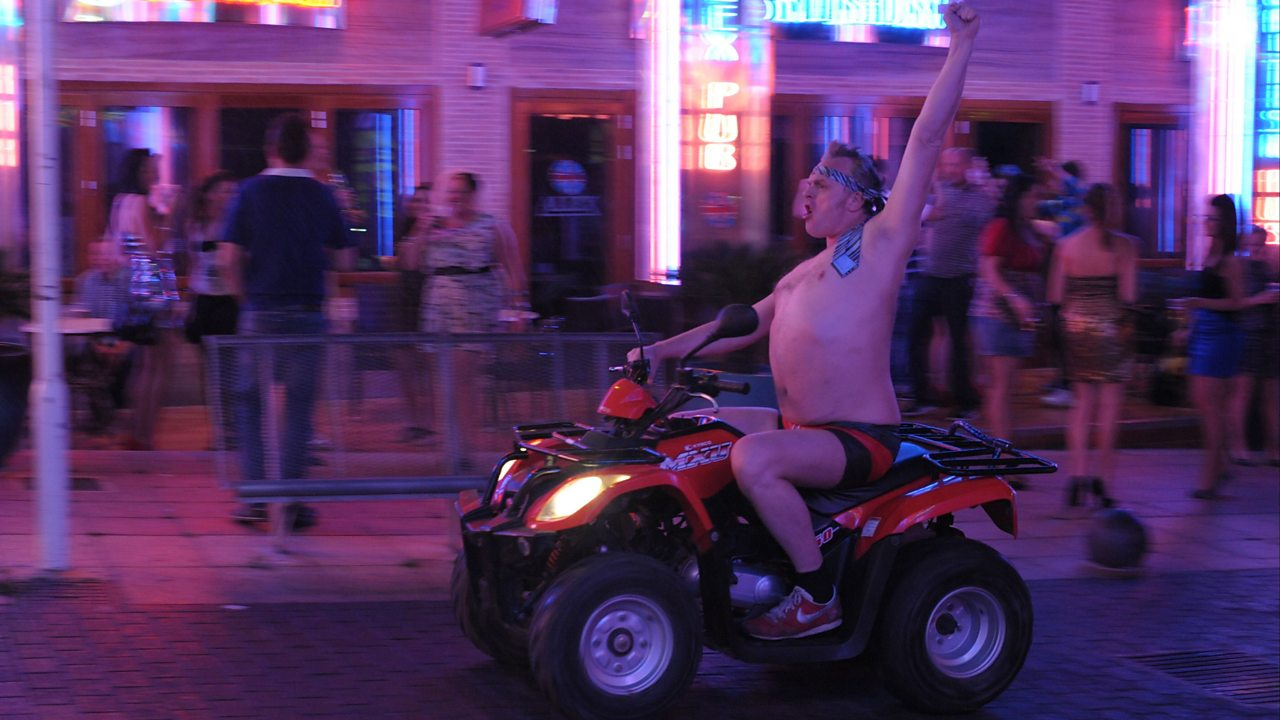Surely a headteacher would get in a lot of trouble for wearing their school's tie half naked on a quad bike in Crete?

The Inbetweeners series (2008-10) was a bit of an alternative take on school life, and Ben Palmer’s spin-off film adopted a very similar tone. First of all, Will McKenzie (Simon Bird) flagrantly flounces Rudge Park Comprehensive’s uniform policy by wearing a black blazer throughout the series and movie, instead of the the school's regulation blue jumper. It’s also hard to believe that headteacher Mr Gilbert (played by Greg Davies) would have got away with the sheer amount of bullying levelled at Will, let alone his antics in Crete where the students went on holiday.

## The Kindergarten Teacher (2018)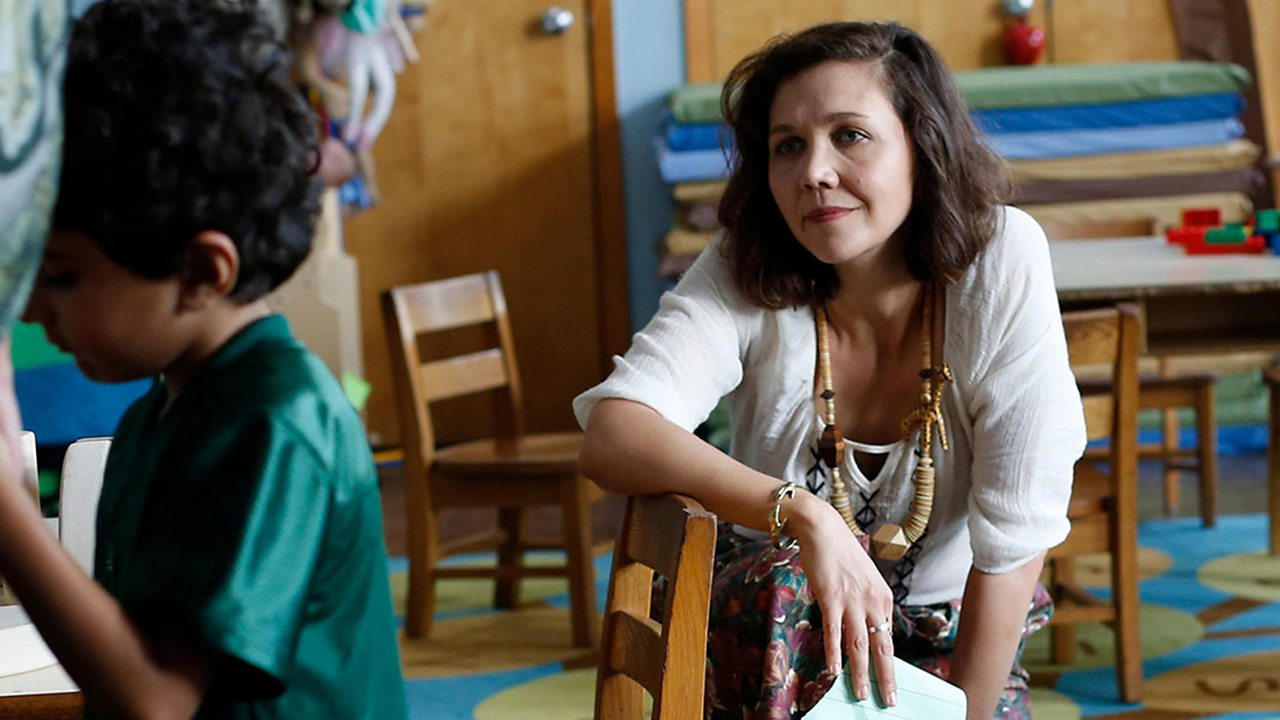"Talent is so fragile and so rare."

Teacher Lisa Spinelli (Maggie Gyllenhaal) is convinced that five year-old Jimmy Roy (Parker Sevak) is a poetic genius. Looking to exploit this, she keeps taking him out of lessons and nap time to talk to him privately, in the hope he'll recite more verses that she can pass off as her own work at evening class. Yet nobody seems to notice this inappropriate behaviour and, even if they do, they don't intervene, with the result that Lisa takes the boy to an open mic event in New York without parental permission.

"),e.close(),u=e.F;r--;)delete u.prototype[i[r]];return u()};e.exports=Object.create||function(e,t){var n;return null!==e?(l.prototype=r(e),n=new l,l.prototype=null,n[o]=e):n=u(),void 0===t?n:a(n,t)}},function(e,t,n){var r=n(1),a=n(11),i=n(19);e.exports=n(2)?Object.defineProperties:function(e,t){a(e);for(var n,o=i(t),l=o.length,u=0;l>u;)r.f(e,n=o[u++],t[n]);return e}},function(e,t,n){var r=n(0).document;e.exports=r&&r.documentElement},function(e,t,n){var r=n(7),a=n(34).f,i={}.toString,o="object"==typeof window&&window&&Object.getOwnPropertyNames?Object.getOwnPropertyNames(window):[],l=function(e){try{return a(e)}catch(e){return o.slice()}};e.exports.f=function(e){return o&&"[object Window]"==i.call(e)?l(e):a(r(e))}},function(e,t,n){var r=n(21),a=n(18),i=n(7),o=n(16),l=n(3),u=n(26),s=Object.getOwnPropertyDescriptor;t.f=n(2)?s:function(e,t){if(e=i(e),t=o(t,!0),u)try{return s(e,t)}catch(e){}if(l(e,t))return a(!r.f.call(e,t),e[t])}},function(e,t,n){"use strict";function r(e){return(r="function"==typeof Symbol&&"symbol"==typeof Symbol.iterator?function(e){return typeof e}:function(e){return e&&"function"==typeof Symbol&&e.constructor===Symbol&&e!==Symbol.prototype?"symbol":typeof e})(e)}function a(e,t){if(!(e instanceof t))throw new TypeError("Cannot call a class as a function")}function i(e,t){for(var n=0;n"),t.close(),c=t.F;r--;)delete c.prototype[i[r]];return c()};t.exports=Object.create||function(t,e){var n;return null!==t?(a.prototype=r(t),n=new a,a.prototype=null,n[u]=t):n=c(),void 0===e?n:o(n,e)}},function(t,e,n){var r=n(75),o=n(124),i=o?function(t,e){return o.set(t,e),t}:r;t.exports=i},function(t,e,n){var r=n(125),o=r&&new r;t.exports=o},function(t,e,n){var r=n(17),o=n(1),i=r(o,"WeakMap");t.exports=i},function(t,e,n){(function(e){var n="object"==typeof e&&e&&e.Object===Object&&e;t.exports=n}).call(e,n(47))},function(t,e){function n(t){if(null!=t){try{return o.call(t)}catch(t){}try{return t+""}catch(t){}}return""}var r=Function.prototype,o=r.toString;t.exports=n},function(t,e,n){function r(t,e,n,_,m,x,j,w,O,A){function P(){for(var d=arguments.length,h=Array(d),v=d;v--;)h[v]=arguments[v];if(T)var y=f(P),g=u(h,y);if(_&&(h=o(h,_,m,T)),x&&(h=i(h,x,j,T)),d-=g,T&&d1&&h.reverse(),k&&O0){if(++e>=r)return arguments}else e=0;return t.apply(void 0,arguments)}}var r=800,o=16,i=Date.now;t.exports=n},function(t,e,n){function r(t,e,n){var r=e+"";return u(t,i(r,a(o(r),n)))}var o=n(233),i=n(234),u=n(137),a=n(237);t.exports=r},function(t,e,n){var r=n(235),o=n(135),i=o(r);t.exports=i},function(t,e,n){var r=n(17),o=function(){try{var t=r(Object,"defineProperty");return t({},"",{}),t}catch(t){}}();t.exports=o},function(t,e){function n(t){return t.placeholder}t.exports=n},function(t,e,n){function r(t){var e=o(t),n=e%1;return e===e?n?e-n:e:0}var o=n(246);t.exports=r},function(t,e,n){function r(t,e){return t&&o(e,i(e),t)}var o=n(51),i=n(31);t.exports=r},function(t,e,n){function r(t,e,n){var r=t[e];a.call(t,e)&&i(r,n)&&(void 0!==n||e in t)||o(t,e,n)}var o=n(85),i=n(86),u=Object.prototype,a=u.hasOwnProperty;t.exports=r},function(t,e,n){function r(t,e){var n=u(t),r=!n&&i(t),s=!n&&!r&&a(t),p=!n&&!r&&!s&&f(t),d=n||r||s||p,h=d?o(t.length,String):[],v=h.length;for(var y in t)!e&&!l.call(t,y)||d&&("length"==y||s&&("offset"==y||"parent"==y)||p&&("buffer"==y||"byteLength"==y||"byteOffset"==y)||c(y,v))||h.push(y);return h}var o=n(248),i=n(87),u=n(2),a=n(88),c=n(83),f=n(144),s=Object.prototype,l=s.hasOwnProperty;t.exports=r},function(t,e,n){var r=n(251),o=n(90),i=n(91),u=i&&i.isTypedArray,a=u?o(u):r;t.exports=a},function(t,e,n){function r(t){if(!o(t))return i(t);var e=[];for(var n in Object(t))a.call(t,n)&&"constructor"!=n&&e.push(n);return e}var o=n(92),i=n(252),u=Object.prototype,a=u.hasOwnProperty;t.exports=r},function(t,e){function n(t,e){return function(n){return t(e(n))}}t.exports=n},function(t,e,n){function r(t){return null!=t&&i(t.length)&&!o(t)}var o=n(76),i=n(89);t.exports=r},function(t,e,n){function r(t,e,n,R,C,M){var W,L=e&A,D=e&P,B=e&k;if(n&&(W=C?n(t,R,C,M):n(t)),void 0!==W)return W;if(!j(t))return t;var F=_(t);if(F){if(W=y(t),!L)return s(t,W)}else{var N=v(t),q=N==S||N==T;if(m(t))return f(t,L);if(N==I||N==E||q&&!C){if(W=D||q?{}:b(t),!L)return D?p(t,c(W,t)):l(t,a(W,t))}else{if(!z[N])return C?t:{};W=g(t,N,L)}}M||(M=new o);var U=M.get(t);if(U)return U;if(M.set(t,W),w(t))return t.forEach(function(o){W.add(r(o,e,n,o,t,M))}),W;if(x(t))return t.forEach(function(o,i){W.set(i,r(o,e,n,i,t,M))}),W;var \$=B?D?h:d:D?keysIn:O,G=F?void 0:\$(t);return i(G||t,function(o,i){G&&(i=o,o=t[i]),u(W,i,r(o,e,n,i,t,M))}),W}var o=n(93),i=n(82),u=n(142),a=n(141),c=n(276),f=n(279),s=n(50),l=n(280),p=n(282),d=n(152),h=n(283),v=n(32),y=n(287),g=n(288),b=n(293),_=n(2),m=n(88),x=n(294),j=n(11),w=n(296),O=n(31),A=1,P=2,k=4,E="[object Arguments]",S="[object Function]",T="[object GeneratorFunction]",I="[object Object]",z={};z[E]=z["[object Array]"]=z["[object ArrayBuffer]"]=z["[object DataView]"]=z["[object Boolean]"]=z["[object Date]"]=z["[object Float32Array]"]=z["[object Float64Array]"]=z["[object Int8Array]"]=z["[object Int16Array]"]=z["[object Int32Array]"]=z["[object Map]"]=z["[object Number]"]=z[I]=z["[object RegExp]"]=z["[object Set]"]=z["[object String]"]=z["[object Symbol]"]=z["[object Uint8Array]"]=z["[object Uint8ClampedArray]"]=z["[object Uint16Array]"]=z["[object Uint32Array]"]=!0,z["[object Error]"]=z[S]=z["[object WeakMap]"]=!1,t.exports=r},function(t,e,n){function r(t){return u(t)?o(t,!0):i(t)}var o=n(143),i=n(277),u=n(147);t.exports=r},function(t,e){function n(){return[]}t.exports=n},function(t,e,n){var r=n(97),o=n(98),i=n(96),u=n(150),a=Object.getOwnPropertySymbols,c=a?function(t){for(var e=[];t;)r(e,i(t)),t=o(t);return e}:u;t.exports=c},function(t,e,n){function r(t){return o(t,u,i)}var o=n(153),i=n(96),u=n(31);t.exports=r},function(t,e,n){function r(t,e,n){var r=e(t);return i(t)?r:o(r,n(t))}var o=n(97),i=n(2);t.exports=r},function(t,e,n){var r=n(1),o=r.Uint8Array;t.exports=o},function(t,e,n){function r(t){return"function"==typeof t?t:null==t?u:"object"==typeof t?a(t)?i(t,t):o(t):c(t)}var o=n(303),i=n(316),u=n(75),a=n(2),c=n(324);t.exports=r},function(t,e,n){function r(t,e,n,u,a){return t===e||(null==t||null==e||!i(t)&&!i(e)?t!==t&&e!==e:o(t,e,n,u,r,a))}var o=n(305),i=n(3);t.exports=r},function(t,e,n){function r(t,e,n,r,f,s){var l=n&a,p=t.length,d=e.length;if(p!=d&&!(l&&d>p))return!1;var h=s.get(t);if(h&&s.get(e))return h==e;var v=-1,y=!0,g=n&c?new o:void 0;for(s.set(t,e),s.set(e,t);++v

p))return!1;var h=s.get(t);if(h&&s.get(e))return h==e;var v=-1,y=!0,g=n&c?new o:void 0;for(s.set(t,e),s.set(e,t);++v